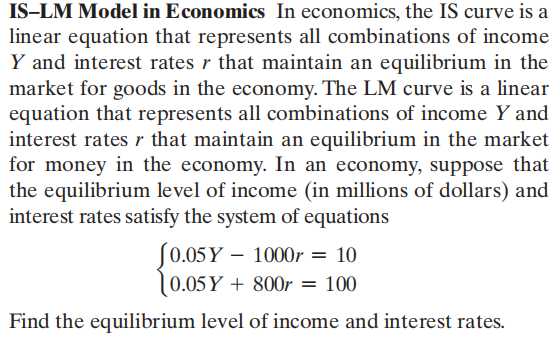### Still have math questions?

Algebra
QuestionIS-LM Model in Economics In economics, the IS curve is a linear equation that represents all combinations of income

$$Y$$ and interest rates $$r$$ that maintain an equilibrium in the market for goods in the economy. The LM curve is a linear equation that represents all combinations of income $$Y$$ and interest rates $$r$$ that maintain an equilibrium in the market for money in the economy. In an economy, suppose that the equilibrium level of income (in millions of dollars) and interest rates satisfy the system of equations

$$\left\{ \begin{array} { l } { 0.05 Y - 1000 r = 10 } \\ { 0.05 Y + 800 r = 100 } \end{array} \right.$$

Find the equilibrium level of income and interest rates.

$$(Y,r) = (1200,\frac{1}{20})$$